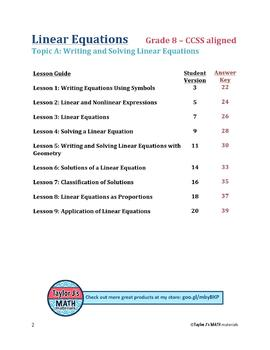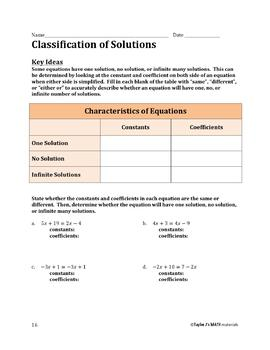# Writing and Solving Linear Equations Bundle7th - 10th
Subjects
Standards
Resource Type
Formats Included
• Zip
•Activity
Pages
38 pages
\$11.47
Bundle
List Price:
\$12.75
You Save:
\$1.28
\$11.47
Bundle
List Price:
\$12.75
You Save:
\$1.28
Easel Activities Included
Some resources in this bundle include ready-to-use interactive activities that students can complete on any device. Easel by TpT is free to use! Learn more.

### Description

Students practice writing and solving equations in this 9 pack bundle!

**If you only need one of the lessons, each is sold individually!

What is covered:

- It reviews the following vocabulary and key terms: coefficient, term, linear, nonlinear, equation, and types of angles

Worksheet Breakdown:

1. Writing Equations Using Symbols Worksheet: Students practice writing equations from words

2. Linear and Nonlinear Expressions Worksheet: Students write an expression from words and then determine whether it is linear or nonlinear

3. Linear Equations Worksheet: Students determine if given values are solutions to equations

4. Solving a Linear Equation Worksheet: Students solve one-step and two-step equations

5. Writing and Solving Linear Equations with Geometry Worksheet: Students practice writing and solving equations based on geometry word problems

6. Solutions of a Linear Equation Worksheet: Students practice solving equations where distributive property is needed; no solution problems are included

7. Classifications of Solutions Worksheet: Students simplify equations and determine if they have one, no, or infinite solutions

8. Linear Equations as Proportions Worksheet: Students solve equations by multiplying each numerator with the other sides denominator

9. Application of Linear Equations Worksheet: Students write and solve equations from words

Possible uses:

- End-of-topic cumulative review guide

- Extra practice for struggling students

- Homework

- Test prep

*************************************************************************************************************

You might be interested in:

- these FREEBIES

- the unit on Congruence

- the unit on Linear Equations

- the unit on Percents & Proportional Relationships

or my store for other material to supplement the 7th & 8th grade curriculum!

Follow My Store to receive email updates on new items, product launches, and sales!

**If you have any requests and/or updates for my work, please message me!

Total Pages
38 pages
N/A
Teaching Duration
N/A
Report this resource to TpT
Reported resources will be reviewed by our team. Report this resource to let us know if this resource violates TpT’s content guidelines.

### Standards

to see state-specific standards (only available in the US).
Solve linear equations in one variable.

### Questions & Answers

Teachers Pay Teachers is an online marketplace where teachers buy and sell original educational materials.